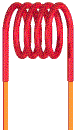# Electronics - Magnetism and Electromagnetism

### Exercise :: Magnetism and Electromagnetism - Filling the Blanks

1.When the current through a coil reverses direction, the magnetic field around the coil _____.

 A. does not change B. disappears C. increases in strength D. reverses direction

Explanation:

No answer description available for this question. Let us discuss.

2.

The flux density is ___ when the magnetic flux is 9 µWb and the cross-sectional area of the magnetic field is 5 × 10–3 m2.

 A. 1800 µT B. 555.56 T C. 9 µT D. 5 × 10–3 T

Explanation:

No answer description available for this question. Let us discuss.

3.

After the magnetizing force has been removed, the ratio of the remaining magnetic flux to the saturated magnetic flux is called _____________.

 A. saturation B. retentivity C. hysteresis D. coercivity

Explanation:

No answer description available for this question. Let us discuss.

4.

You can determine the direction of the lines of force surrounding a conductor by using _____.

 A. the right-hand rule B. the left-hand rule C. Lenz's law D. Faraday's law

Explanation:

No answer description available for this question. Let us discuss.

5.

A group of force lines going from the north pole to the south pole of a magnet is called the magnetic ___.

 A. retentivity B. reluctance C. hysteresis D. flux